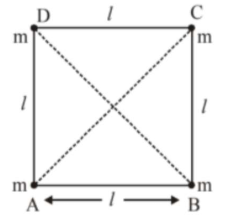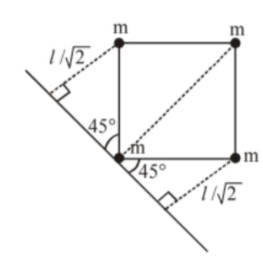# Four equal masses, m each are placed at the corners of a square of length

Question:

Four equal masses, $\mathrm{m}$ each are placed at the corners of a square of length

$(l)$ as shown in the figure. The moment of inertia of the system about an axis passing through $\mathrm{A}$ and parallel to $\mathrm{DB}$ would be :1. $\mathrm{m} / 2$

2. $2 \mathrm{ml}^{2}$

3. $3 \mathrm{ml}^{2}$

4. $\sqrt{3} \mathrm{ml}^{2}$

Correct Option: , 3

Solution:

(3)

Moment of inertia of point mass

$=$ mass $\times(\text { Perpendicular distance from axis })^{2}$Moment of Inertia

$=\mathrm{m}(0)^{2}+\mathrm{m}(l \sqrt{2})^{2}+\mathrm{m}\left(\frac{l}{\sqrt{2}}\right)^{2}+\mathrm{m}\left(\frac{l}{\sqrt{2}}\right)^{2}$

$=3 \mathrm{~m} /{ }^{2}$